Download Presentation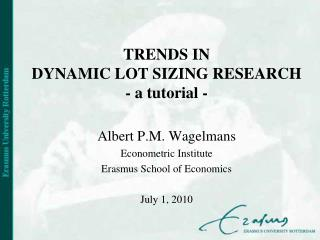TRENDS IN DYNAMIC LOT SIZING RESEARCH - a tutorial -

# TRENDS IN DYNAMIC LOT SIZING RESEARCH - a tutorial - - PowerPoint PPT Presentation

TRENDS IN DYNAMIC LOT SIZING RESEARCH - a tutorial -. Albert P.M. Wagelmans Econometric Institute Erasmus School of Economics July 1, 2010. Turkish football supporters in Holland (2008). Management Science , October 1958, Volume 5, Number 1, pp. 89-96. 592 citations.I am the owner, or an agent authorized to act on behalf of the owner, of the copyrighted work described.
Download Presentation## TRENDS IN DYNAMIC LOT SIZING RESEARCH - a tutorial -

An Image/Link below is provided (as is) to download presentation

Download Policy: Content on the Website is provided to you AS IS for your information and personal use and may not be sold / licensed / shared on other websites without getting consent from its author.While downloading, if for some reason you are not able to download a presentation, the publisher may have deleted the file from their server.

- - - - - - - - - - - - - - - - - - - - - - - - - - E N D - - - - - - - - - - - - - - - - - - - - - - - - - -
Presentation Transcript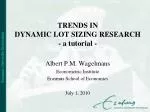### TRENDS IN DYNAMIC LOT SIZING RESEARCH- a tutorial -

Albert P.M. Wagelmans

Econometric Institute

Erasmus School of Economics

July 1, 2010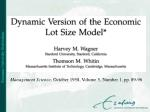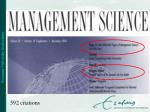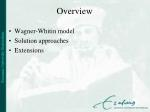Overview

Wagner-Whitin model

Solution approaches

Extensions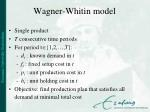Wagner-Whitin model
• Single product
• T consecutive time periods
• For period t{1,2,…,T}:
• dt : known demand in t
• ft : fixed setup cost in t
• pt : unit production cost in t
• ht : unit holding cost in t
• Objective: find production plan that satisfies all demand at minimal total costWagner-Whitin model
• MIP formulation
• Variables for period t:
• xt : number of items produced
• yt : binary variable to indicate a setup (=1) or not (=0)
• It : ending inventory levelWagner-Whitin model

MIP formulation:Wagner-Whitin model
• Notes:
• The original W-W model had stationary costs (and therefore no unit production costs)
• The inventory variables can be substituted out of the model, resulting in a model without holding costs, but with modified unit production costs rtSolutions approaches
• Dynamic programming
• Heuristics
• Mixed integer linear programmingDynamic programming
• Crucial observation: there exists an optimal production plan such that for every period t: It-1 = 0 or xt = 0 (zero-inventory property)
• This means that an optimal production plan is completely determined by its set of production periods
• F(t): optimal value of the lot sizing problem for the first t periodsDynamic programming

Complexity: O(T2)

Refinements to bring down the (practical) running time

Planning horizon theorem (W&W): when the cost coefficients are stationary, then the optimal last production period for the first t+1 periods is not earlier than the optimal last production period for the first t periodsDynamic programming
• Improved DP approaches (Federgruen & Tzur, Wagelmans et al., Aggarwal & Park, early 90s): O(T log T); linear for special cases (incl. stationary costs)
• Geometric technique:Heuristics
• Motivation:
• W&W algorithm too complicated
• W&W algorithm leads to nervousness when implemented in a rolling horizon context
• Heuristics may outperform W&W algorithm in a rolling horizon context
• Assume stationary costs (ignore unit production costs)Heuristics
• Example Silver-Meal:
• Let C(t) denote the cost of producing in period 1 for the first t periods, i.e.,
• Let t* be the first period for which
• Produce in period 1 for the first t* periods
• Continue in a similar way with period t*+1; and so onHeuristics
• Numerous other heuristics: Least unit cost, Part period balancing, Lot for lot,…
• Single pass or with look-back/look-ahead feature to marginally adjust tentative lot sizes
• Many computational studies
• Approximation results (Axsäter, Bitran et al., early 80s)Heuristics
• Examples of approximation results:
• Worst case ratio of Part period balancing is 2
• Worst case performance of the Silver-Meal heuristic can be arbitrarily bad
• Axsäter, 1985: for a large class of lot sizing heuristics, including all well-known single pass heuristics, have worst case ratio at least 2Heuristics

Van den Heuvel & Wagelmans, 2010: any online heuristic has worst case ratio at least 2

Result also holds if we allow look-back/look-ahead for at most a fixed number of periodsHeuristics

W&W algorithm may perform badly in a rolling horizon context because end-of-horizon effects (IT= 0)

Stadtler, 2000: forecast demand beyond T

Fisher et al., 2001: ending inventory evaluation

Van den Heuvel & Wagelmans, 2005: straightforward application of W&W algorithm to extended horizon with demand forecastsHeuristics

Average percentage deviation from optimality

AR: average rankMixed integer programming
• Natural MIP formulation:
• May have fractional LP relaxation; often large integrality gapMixed integer programming

Simple plant location formulation: disaggregate xt into variables xti denoting the amount produced in period t to satisfy demand in periods i ≥ t

The LP relaxation of this formulation has an integer optimal solution (Krarup & Bilde, 1977)Mixed integer programming

LP relaxation of the natural MIP formulation has an integer optmal solution if we add the (m,S) inequalities (Barany et al., 1984):

In other words, we have obtained a complete linear (polyhedral) description of the convex hull of feasible solutionsMixed integer programming

Shortest path network for T = 4

Mij: cost of producing in period i for period i through j

Shortest path (flow) formulation:Extensions
• Backlogging
• Multi-echelon
• Production capacities
• Price dependent demand
• Product returns
• Also: lost sales, minimum order quantities, set-up time, bounds on inventory, different cost functions, perishable goods, product substitution, time windows, multiple items, etcetera, etcetera, ...Backlogging
• Zangwill, 1966/69: concave costs; optimal solutions correspond to extreme flows in certain networksBacklogging
• Dynamic programming based on the property that every production period t produces exactly Dij for some i and j with i ≤ t ≤ j
• O(T2) running time for general concave costs; O(T log T) for setup + linear production costs and linear holding and backlogging costsProduction capacities
• Florian & Klein, 1971: concave costs; between any two consecutive periods with zero inventory, there is at most one production period which produces below its capacity
• Florian et al., 1980: lot-sizing with production capacities is NP-hard, in general
• Stationary capacities: O(T4) DP algorithm
• Stationary capacities and linear holding costs: O(T3) DP algorithm (Van Hoesel & W, 1996)Production capacities
• Solution approaches for non-stationary capacities:
• DP algorithms
• MIP
• ApproximationProduction capacities
• DP algorithms:
• Florian et al., 1980: straightforward DP for general cost functions with variables Ft(It) denoting the minimal cost in the first t periods to attain ending inventory It; O(C1TD1T)running time, where C1T denotes total capacity over the horizon
• Shaw &W, 1998: O(qD1T) algorithm for piecewise linear production costs and general holding costs, where q is the total number of pieces in the production cost functionsProduction capacities
• DP algorithms (cont’d):
• Chen et al., 1994: piecewise linear costs (geometric techniques)Production capacities
• MIP approaches; tight LP bounds through:
• Extending the formulation (simple plant location formulation)
• Reformulation (shortest path, Eppen & Martin, 1987)
• Polyhedral description (Wolsey and others)Management Science, December 2002, Volume 48 ,  Issue 12,  pp. 1587 - 1602Production capacities
• Approximation:
• Van Hoesel & W, 2001: FPTAS for capacitated problem with backlogging and general monotone cost functions
• Based on DP approach with “dual” variables

Gt(b): maximum value of It , which can be achieved by production in the first t periods if the total cost incurred in these periods is at most b

• Chubanov et al, 2006: FPTAS based on standard DP approachMulti-echelon
• Zangwill, 1969: concave costs, no capacities; O(LT4) DP algorithmMulti-echelon
• Van Hoesel et al., 2005: concave costs, stationary production capacities; O(LT2L+3) DP algorithm; better running times for more specific cost functionsPrice dependent demand
• Kunreuther & Schrage, 1973:

where αt,βt≥ 0 en δ(p) non-increasing in p

• Find p* between pl and pu that maximizes profit
• K&S give a local improvement procedure that is not guaranteed to find p* (but often does)
• Van den Heuvel & W, 2006: polynomial method to find p*
• Geunes et al, 2009: generalization to stationary capacitiesProduct returns
• Teunter et al., 2006: dynamic lot sizing with product returns and remanufacturing; stationary costs
• Minimize the total cost composed of holding cost for returns and (re)manufactured products and set-up costs
• Distinguish between joint setup cost for manufacturing and remanufacturing and separate set-up costs (single vs. dedicated production lines)
• O(T4) DP algorithm for joint setup cost case
• Conjecture NP hardness of separate setup cost caseProduct returns
• Retel Helmrich et al, 2010: same model, but with non-stationary costs
• Both cases are NP-hard; seperate setup case is even NP-hard with stationary costs
• Comparison of different MIP formulationsConcluding remarks
• After more than half a century, dynamic lot sizing is still a thriving research area
• Academic research has lead to practical methods for real-life problems
• THANK YOU!